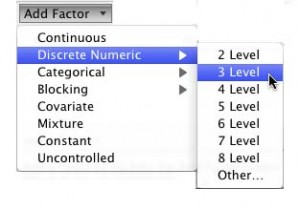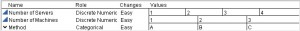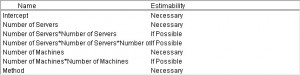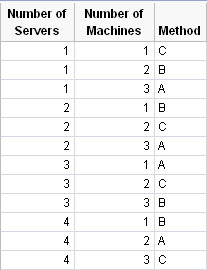Our World Statistics Day conversations have been a great reminder of how much statistics can inform our lives. Do you have an example of how statistics has made a difference in your life? Share your story with the Community!
Choose Language Hide Translation Bar
New in JMP 10 DOE: Discrete Numeric Factors

Among other kinds of factors, the Custom Designer in JMP has facilities for continuous factors and for categorical factors with an arbitrary number of levels. The designer assumes that continuous factors can take any value within the specified range from low to high.

Sometimes, though, there are practical restrictions to the values that a numeric factor can take. For example, in a simulation of a service queue, the number of servers can only realistically take positive integer values like 1, 2 or 3.

Couldn’t you just define a categorical factor with three levels?

Yes, previously one workaround for the above situation was to use a categorical factor with the desired number of levels. This is not completely satisfactory for a number of reasons:

1) Levels of categorical factors have no order, but we know that 2 is between 1 and 3.

2) A categorical factor with three levels has two degrees of freedom, but we generally prefer to separate these effects in a numeric factor into a linear and quadratic component.

This approach is often workable, but sometimes the optimal design includes values that are not the high, middle and low values.

Introducing Discrete Numeric factors…

These factors occupy a middle ground between completely continuous factors that can take any value on an interval and categorical factors that have a fixed number of unordered levels. A discrete numeric factor has an ordered set of allowable values. These values need not be evenly spaced. For example, you could choose the values 1, 3 and 10 for a discrete numeric factor. This spacing could be useful in cases where you think that the response is a function of the logarithm of the factor value.

How do you create a discrete numeric factor?

Figure 1 shows the interface for adding a factor in the Custom Designer. Discrete Numeric factors are second on the list below Continuous factors and above Categorical factors. The figure also shows that you define a discrete numeric factor by specifying how many levels it has.

After making the three-level choice as shown, the factors list has entries for three numeric values that you can supply.Imagine a print shop where customers have access to between one and four service personnel. The servers can use one to three machines, and each machine has three methods of operation. Figure 2 shows the Factors Table for this example.After defining the factors, you proceed to specifying a model. Figure 3 shows the default model for this scenario.In previous versions of the Custom Designer, the default model included only the main effects of each factor. In Figure 3, there are quadratic and cubic terms for the Number of Servers and Number of Machines factors.

Why has the default model changed, and what does an Estimability of ‘If Possible’ mean?

The extra terms for the discrete numeric factors are essential. Without them, the Custom Designer will choose only the high and low values of each factor. For the optimal design to use three levels of a numeric factor, a quadratic effect is needed. Four-level numeric factors require a cubic model term – it takes four unique settings to determine a cubic polynomial.

Designating these extra terms as If Possible instead of Necessary makes the design optimization problem more like the usual default model containing the main effects only. It also allows for choosing a smaller number of runs than would otherwise be necessary. If you want all the terms to be necessary. then select them and then click inside the Estimability column to make the change.

What happens next?

I created a 12-run design that you can see in Table 1.I sorted the table from left to right so the symmetry of the design is evident. Note that the design uses all possible combinations of Number of Servers and Number of Machines. It also uses all combinations of Number of Servers and Method. Since there are only nine combinations of Number of Machines and Method, three of them are repeated.

The main thing to notice is that for the discrete numeric factors, the design uses only their allowable levels.

Article Labels

There are no labels assigned to this post.

Article Tags